# 阿里大航杯AI电力大赛比赛分享及数加平台，机器学习pai使用经验

+关注继续查看

1. 背景

    本文以阿里大航杯AI电力大赛为背景，讲述数加平台及机器学习pai的使用。大赛聚焦电力市场营销活动业务难点——企业用电需求预测，开放了扬中市高新区1000多家企业的历史用电量数据，要求参赛者通过模型算法精准预测该地区下一个月的每日总用电量。

2. 数据介绍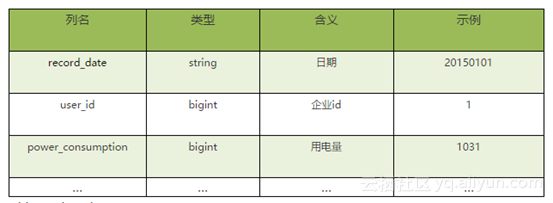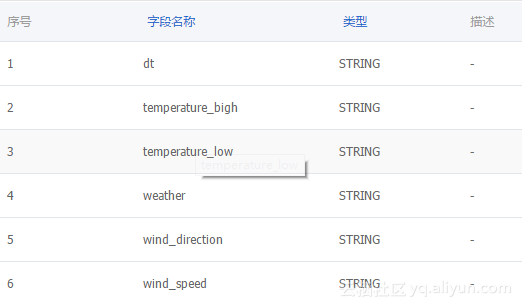3 解决方案概述

3.1特征工程

Record_date,power_consumption,year,month,day,dow,mean_1,d_max_1,d_min_1,d_median_1,mean_2,d_max_2,d_min_2,d_median_2,mean_avg,mean_dist,d_max_avg,d_max_dist,d_min_avg,d_min_dist,d_median_avg,d_median_dist,row_n

tem_h,tem_l,tem_avg,tem_dist

season,holiday

1.在左边首先选择任务开发-->自己建立的文件夹（有多位队员可以创建自己的文件夹，方便使用）-->右击新建任务（这里使用的ODPS_SQL，因为sql最容易上手）-->键入脚本名称即可。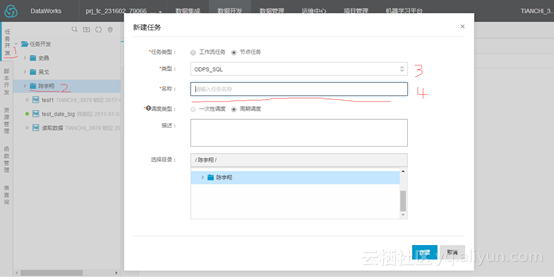2.在编辑框中，编辑自己的sql语句（建表时注意不要与队友所建表名称一致，否则将无法更新表中字段，这里系统是不提示表名是否重复的），然后运行即可。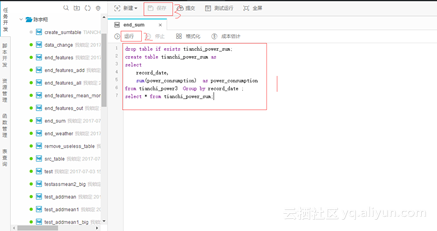Tips: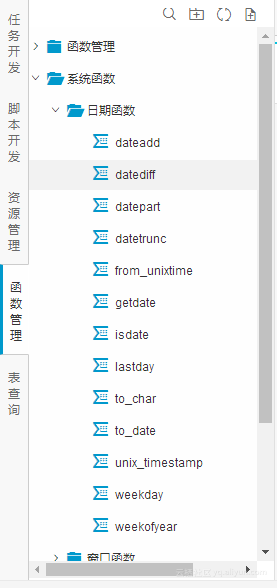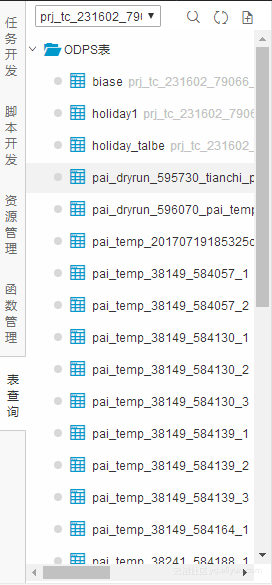3.2模型的设计及融合

Gbdt模型的训练及数据预测

• 实验的创建：
在首页中可以选择从已有实验的模板创建也可新建空白实验，本实验选择新建空白实验：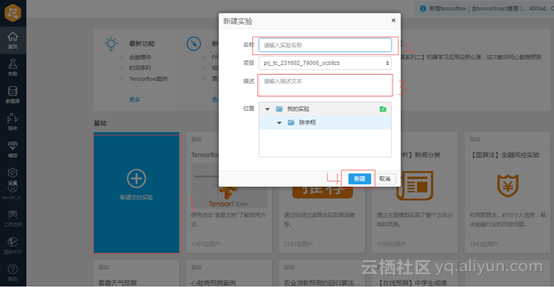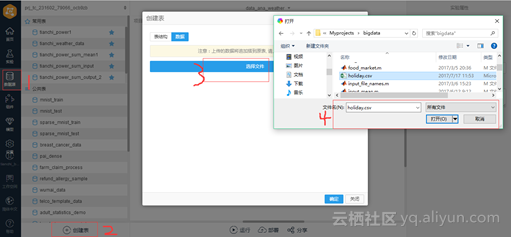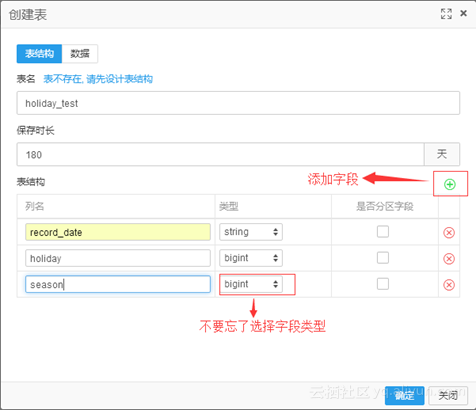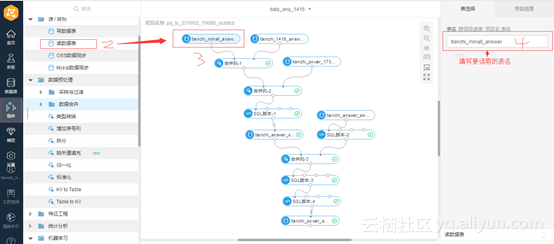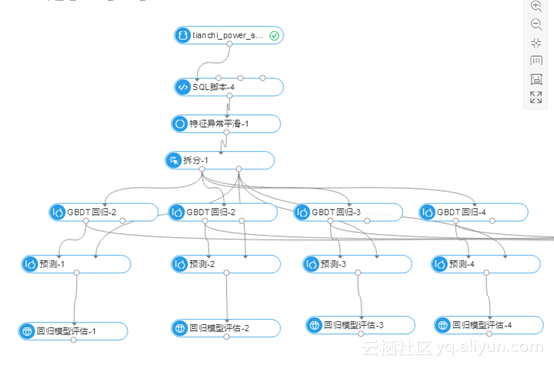select
record_date,

power_consumption,
year,
month,
day,
dow,
mean_1,
d_max_1,
d_min_1,
d_median_1,
mean_2,
d_max_2,
d_min_2,
d_median_2,
mean_avg,
mean_dist,
d_max_avg,
d_max_dist,
d_min_avg,
d_min_dist,
d_median_avg,
d_median_dist,
tem_h,
tem_l,
tem_avg,
tem_dist,
row_n,
holiday,
season

from ${t1} where record_date<='2016-01-30'or record_date>='2016-02-19' gbdt的训练参数（4个模型仅在树的数量，学习率，最大叶子树，树深进行了微调,其它参数一致）：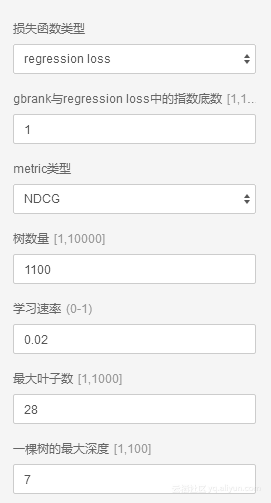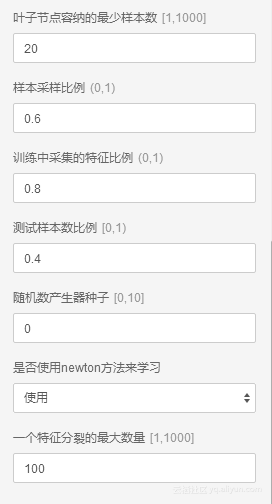本实验gbdt预测部分如下：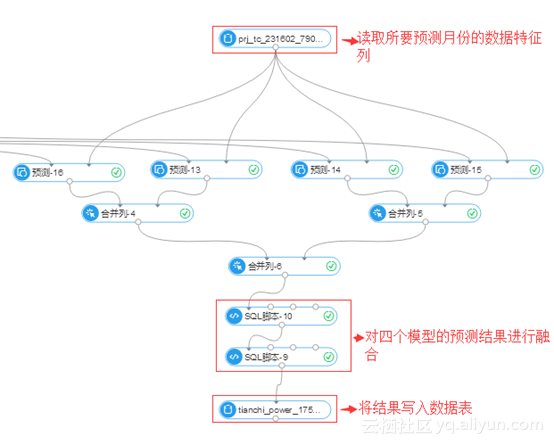对四个模型的预测结果融合部分sql语句： select concat(year,'/',month,'/',day) as predict_date, (prediction_a+prediction_b+prediction_c+prediction_d)/4 as power_mean, prediction_a, prediction_b, prediction_c, prediction_d from${t1} ;

select
predict_date,
(case when abs(prediction_a-power_mean)when abs(prediction_b-power_mean)when abs(prediction_c-power_mean)when abs(prediction_d-power_mean)end) as prediction
from \${t1};

Xgboost 模型的训练及预测

drop table if exists xgb_pred_1;
DROP OFFLINEMODEL IF EXISTS xgboost_1;
-- train
PAI
-name xgboost
-project algo_public
-Deta="0.01"
-Dobjective="reg:linear"
-DitemDelimiter=","
-Dseed="0"
-Dnum_round="3500"
-DlabelColName="power_consumption"
-DinputTableName="tianchi_power_sum_min_input_1"
-DenableSparse="false"
-Dmax_depth="8"
-Dsubsample="0.4"
-Dcolsample_bytree="0.6"
-DmodelName="xgboost_1"
-Dgamma="0"
-Dlambda="50"
-DfeatureColNames="year,month,day,dow,holiday,season,mean_1,d_max_1,d_min_1,d_median_1,mean_2,d_max_2,d_min_2,d_median_2,mean_avg,mean_dist,d_max_avg,d_max_dist,d_min_avg,d_min_dist,d_median_avg,d_median_dist,tem_h,tem_l,tem_avg,tem_dist,row_n"
-Dbase_score="0.11"
-Dmin_child_weight="100"
-DkvDelimiter=":";

-- predict
PAI
-name prediction
-project algo_public
-DdetailColName="prediction_detail"
-DappendColNames="year,day"
-DmodelName="xgboost_1"
-DitemDelimiter=","
-DresultColName="prediction_result"
-Dlifecycle="28"
-DoutputTableName="xgb_pred_1"
-DscoreColName="prediction_score"
-DkvDelimiter=":"
-DfeatureColNames="year,month,day,dow,holiday,season,mean_1,d_max_1,d_min_1,d_median_1,mean_2,d_max_2,d_min_2,d_median_2,mean_avg,mean_dist,d_max_avg,d_max_dist,d_min_avg,d_min_dist,d_median_avg,d_median_dist,tem_h,tem_l,tem_avg,tem_dist,row_n"
-DinputTableName="tianchi_power_sum_min_output_2"
-DenableSparse="false";

select * from xgb_pred_1;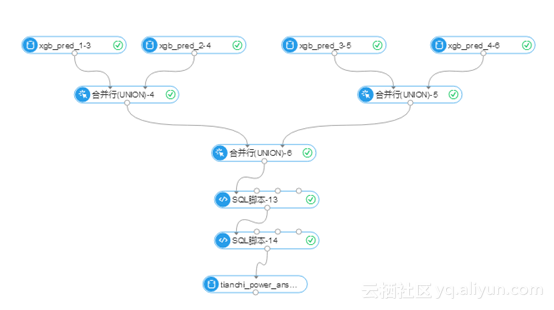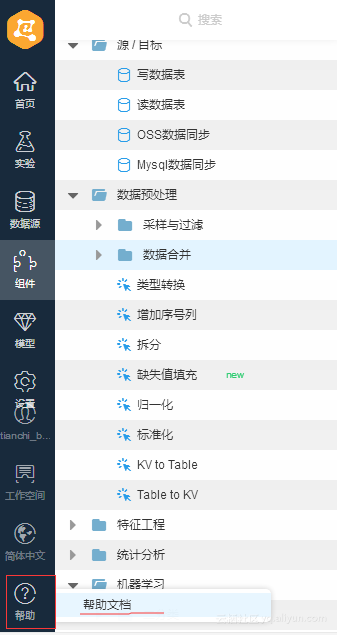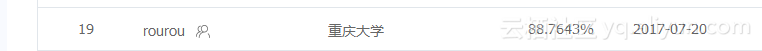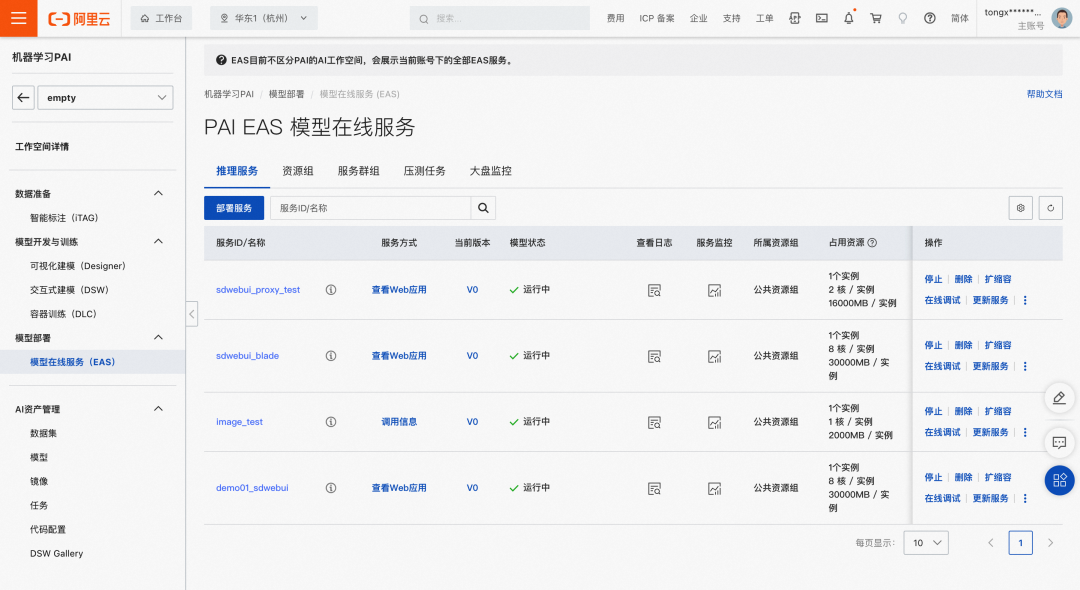11904 0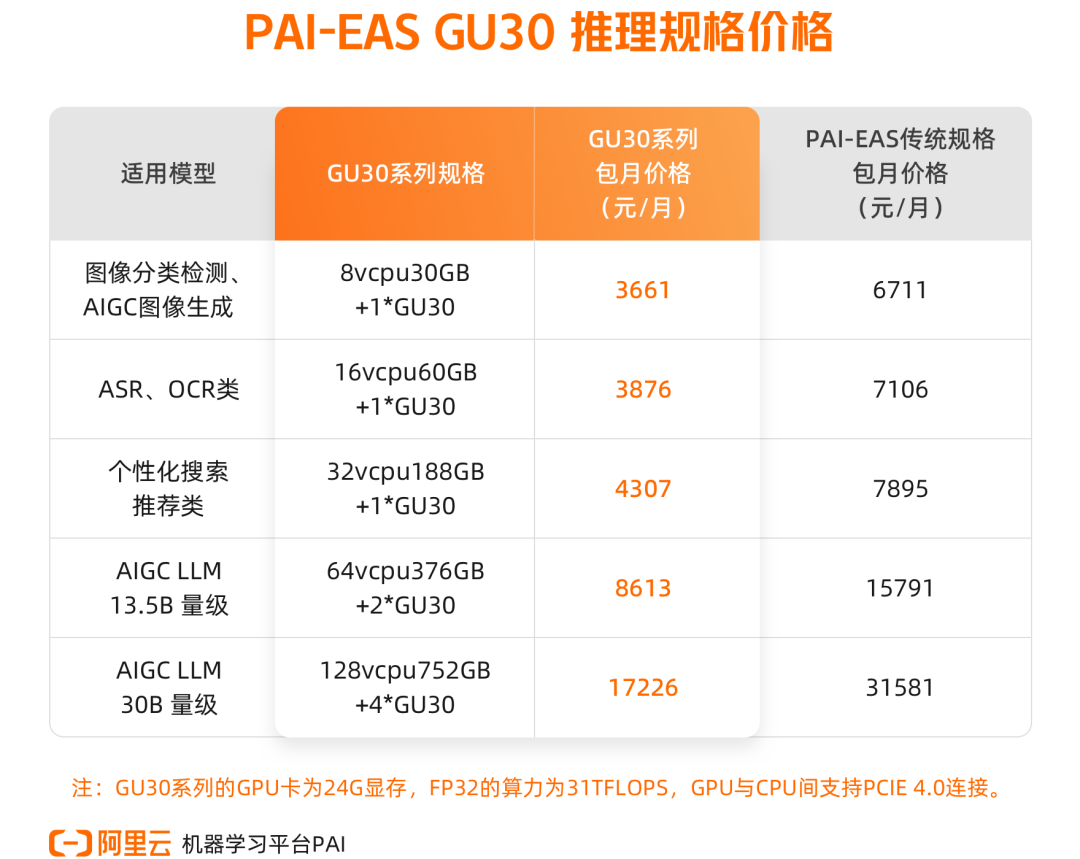AI推理服务平台升级，阿里云机器学习PAI推出新规格

305 0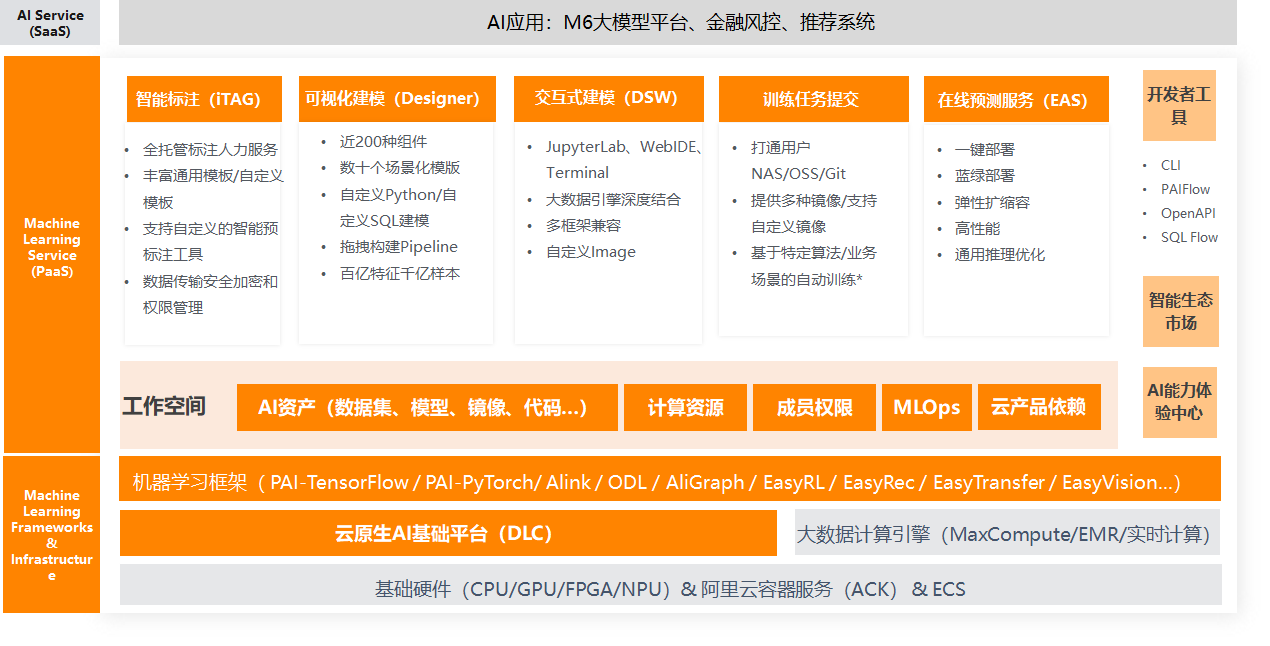397 0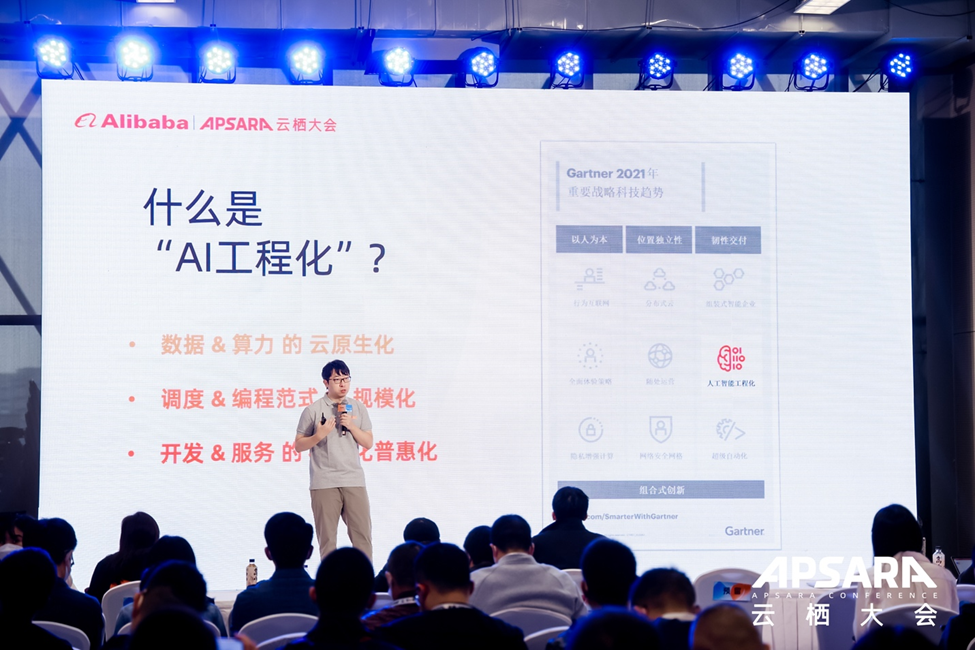672 0PAI AI行业插件OCR识别Quick Start

241 0482 0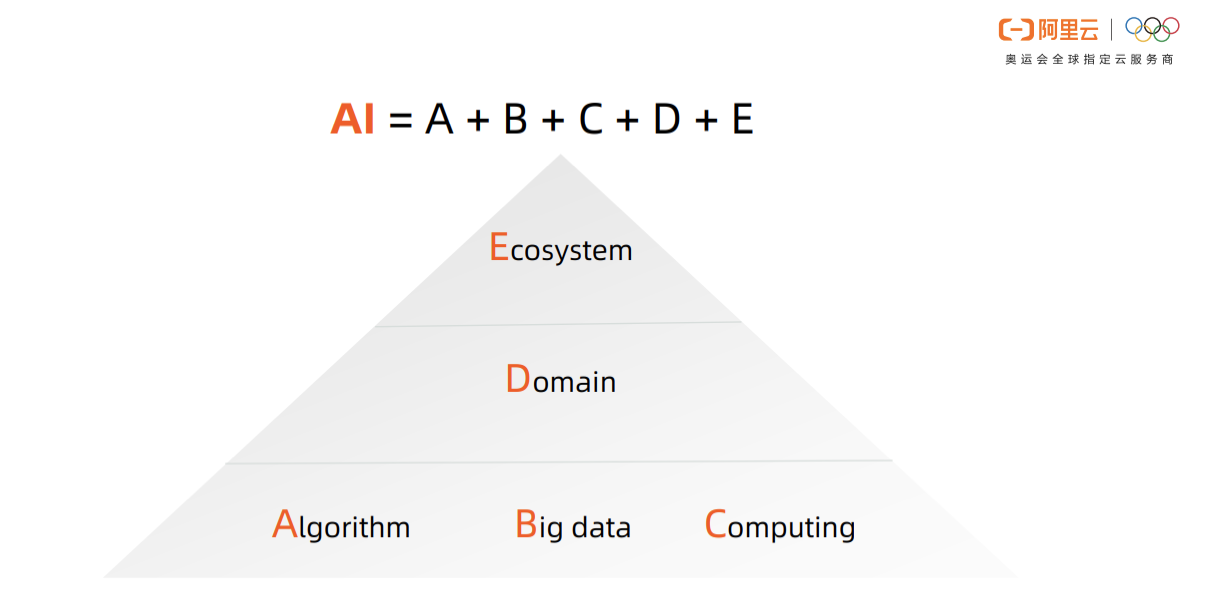PAI：一站式云原生AI平台

3292 0

+ 订阅

AI数学基础1344252